### Sumpner's Test (Back to Back Test)

Sumpner's Test (Back to Back Test)
The Sumpner's test is another method of determining efficiency, regualtion and heating under load conditions. The O.C. and S.C. tests give us the equivalent circuit parameters but ca not give heating information under various load conditions. The Sumpner's test gives heating information also. In O.C. test, there is no load on the transformer while in S.C. circuit test also only fractional load gets applied. In all in O.C. and S.C. tests, the loading conditions are absent. Hence the results are inaccurate. In Sumpner's test, actual loading conditions are simulated hence the results obtained are much more accurate. Thus Sumpner;s test is much improved method of predetermining regulation and efficiency than O.C. and S.C. tests.
The Sumpner's test requires two identical transformers. Both the transformers are connected to the supply such that one transformer is loaded on the other. Thus power taken from the supply is that much necessary for supplying the losses of both the transformers and there is very small loss in the control circuit.
While conducting this test, the primaries of the two identical transformers are connected in parallel across the supply V1. While the secondaries are connected in series opposition so that induced e.m.f.s in the two secondaries oppose each other. The secondaries are supplied from another low voltage supply are connected in each circuit to get the readings. The connection diagram is shown in the Fig. 1.Fig  1  Connection diagram for Sumpner's test

T1 and T2 are two identical transformers. The secondaries of T1 and T2 are connected in series opposition. So EEF = EGH   i.e. induced in two secondaries are equal but the secondaries are connected such that E is connected to G and F is connected to H. Due to such series opposition, two e.m.f.s act in opposite direction to each other and cancel each other. So net voltage ion the local circuit of secondaries is zero, when primaries are excited by supply 1 of rated voltage and frequency. So there is no current flowing in the loop formed by two secondaries. The series opposition can be checked by another voltmeter connected in the secondary circuit as per polarity test. If it reads zero, the secondaries are in series opposition and if it reads double the induced e.m.f. in each secondary, it is necessary to reverse the connections of one of the secondaries.
As per superposition theorem, if V2 is assumed zero then due to phase opposition to current flows through secondary and both the transformers T1, T2 are as good as on no load. So O.C. test gets simulated. The current drawn from source V1 in such case is 2 Io where Io is no load current of each transformer. The input power as measured by wattmeter W1 thus reads the iron losses of both the transformers.
...                       P per transformer =W1 /2           as T1, T2 are identical
Then a small voltage V2 is injected into the secondary with the help of low voltage transformer, by closing the switch S. With regulation mechanism, the voltage V2 is adjusted so that the rated secondary current I flows through the secondaries as shown. I flows from E to F and then from H to G. The flow of I is restricted to the loop B A I J C D L K B and it does not pass through W1. Hence W1 continues to read core losses. Both primaries and secondaries carry rated current so S.C. test condition gets simulated. Thus the wattmeter W2 reads the total full load copper losses of both the transformers.
...               (Pcu) F.L.per transformer = W2 /2
Key Point : Thus in the sumpner's test without supplying the load, full iron loss occurs in the core while full copper loss occurs in the windings simultaneously. Hence heat run test can be conducted on the two transformers. In O.C. and S.C. test, both the losses do not occur simultaneously hence heat run test can not be conducted. This is the advantage of Sumpner's test.
From the test results the full load efficiency of each transformer can be calculated as,

where             output = VA rating x cos Φ2
Key Point : As all the voltage, currents and powers are measured during the test, the equivalent circuit parameters also can be determined. Hence the regulation at any load and load power factor condition can be predetermined.
The only limitation is that two identical transformers are required. In practice exact identical transformers can not be obtained. As two transformers are required, the test is not economical.
Example 1 : Two similar 200 KVA, single phase transformers gave the following results when tested by Sumpner's test :
Mains wattmeter W1 = 4 kW
Series wattmeter W2 = 6 kW at full load current
Find out individual transformer efficiencies at,
i) Full load at unity p.f.     ii) Half load at 0.8 p.f. lead.
Solution : The given values are,
Rating = 200 KVA,       W1 = 4 kW,  W = 6 kW
W1 = Iron loss of the both transformers
...          P = W1/2 = 4/2 = 2 kw for individual transformer
W2 = Full load copper loss for both the transformer
...          (Pcu)F.L. = W2 /2= 6/2 = 3 kW for individual transformer
i) At full load,

ii) At half load, cos Φ2 = 0.8 and n = 1/2 = 0.5

= 96.67%

#### 19 comments:

1.It was very helpfull.. i m so glad that i found this blog..Keep Posting

2.thanks a lot....this information really helped me......thank you very much...

3.This comment has been removed by the author.

4.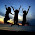5.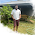very helpfull for those who cannot buy txt buks..... good word dude

6.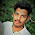If we want to connect load to that circuit, where we want to connect the load?

7.thanx alot now i will shock my teachers tomorrow in lab

8.Easy language than books easily understood .thanks

9.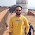10.Thank you...Theory written in my record

11.Very good explanation.

12.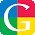Easy language than books easily understood .thanksSumpner's Test or Back to Back Test read here

13.thanks a lot................very helpfull

14.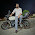can u add back to back test of 3 phase transformer

15.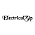easy method to undrstnd,,back to back test or sumpner's test

16.thank u very much
but power factor... is it W2 / V2*I2

17.I am a 2 nd year EEE student I was asked to take a seminar on SUMPNER'S TEST .....this blog was really helpful for me in preparing for my seminar.... It gives me a better idea than sitting in the class for hours and taking notes....... Just within 10 minutes i was able to understand the concept....Thank u very much......

18.##it was very helpful to us..�� thank u..vry Gud explanation..

19.Thank you so much sir.. it was very helpful ..